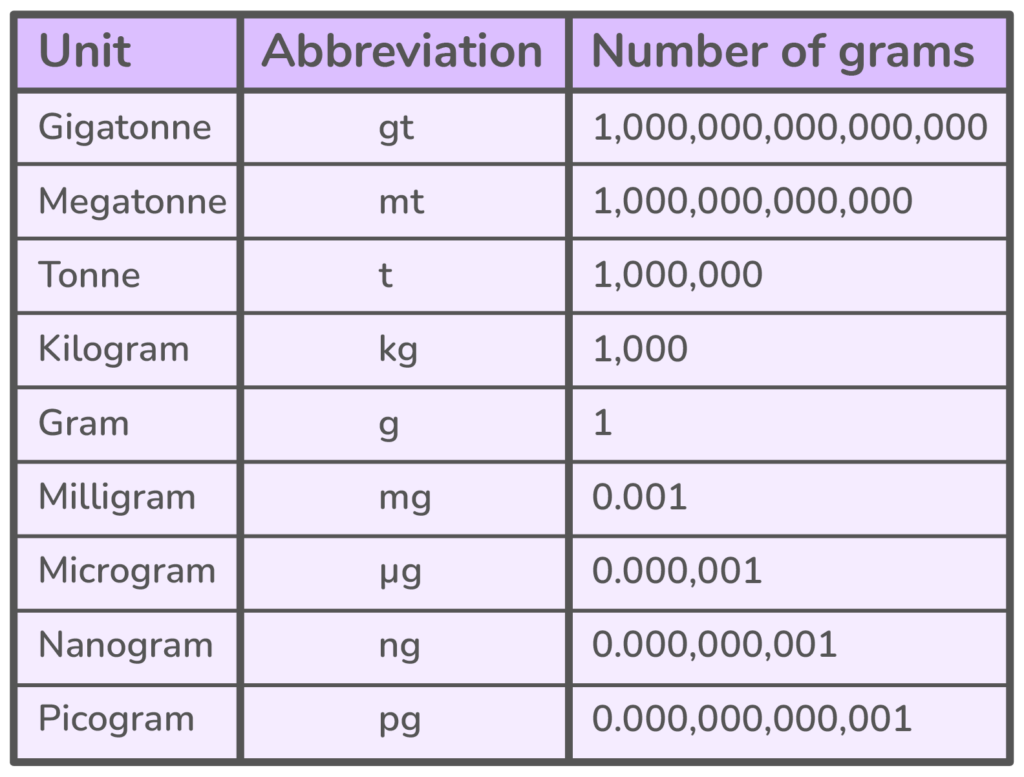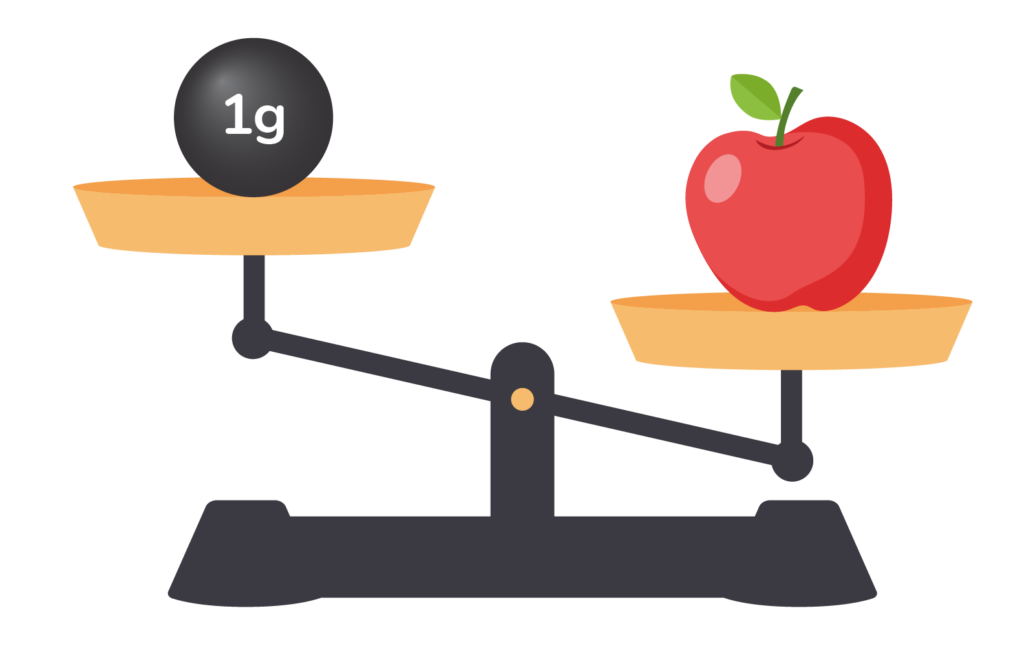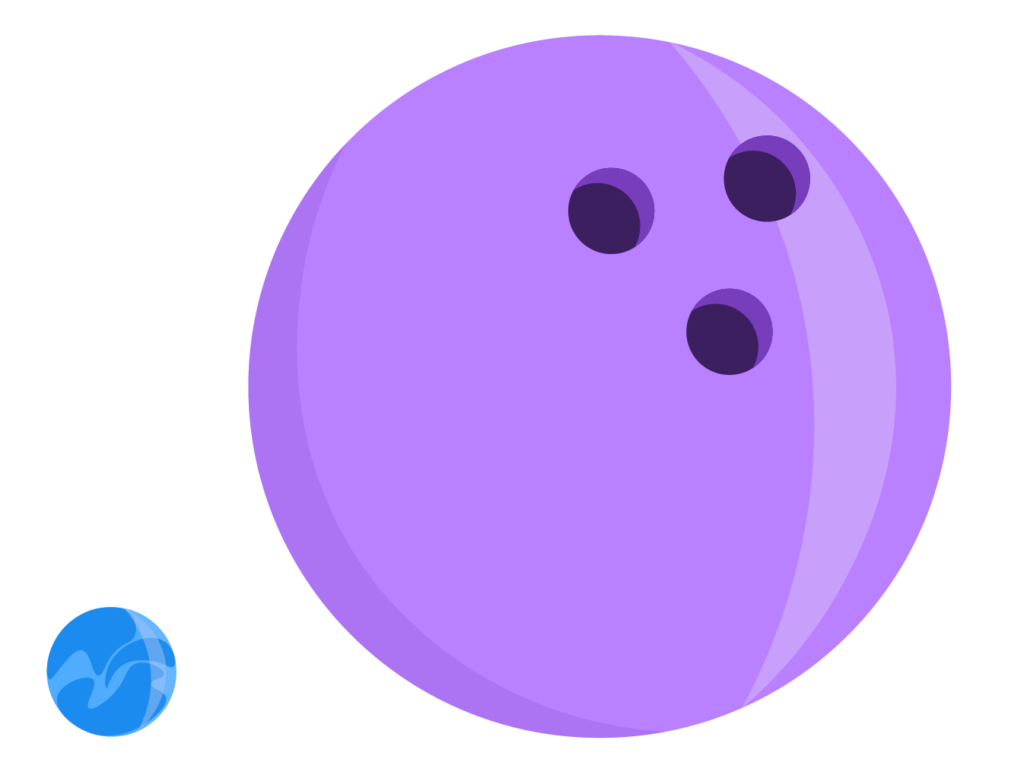# What is Mass? Mass Definition & Examples

Mass simplified: an easy-to-understand and straightforward guide to mass, its definition, how it’s used, and the different ways to calculate itAuthor
Tess LouckaPublished
November 14, 2023# What is Mass? Mass Definition & Examples

Mass simplified: an easy-to-understand and straightforward guide to mass, its definition, how it’s used, and the different ways to calculate itAuthor
Tess LouckaPublished
Nov 14, 2023# What is Mass? Mass Definition & Examples

Mass simplified: an easy-to-understand and straightforward guide to mass, its definition, how it’s used, and the different ways to calculate itAuthor
Tess LouckaPublished
Nov 14, 2023Key takeaways

• Mass is the quantity of matter that makes up a given object
• Mass and weight are not the same thing. Mass deals with matter. Weight deals with the gravitational pull the Earth has on an object.
• The formula for calculating mass is Mass = Density x Volume. You can also find an object’s inertial and gravitational mass.

Mass is everywhere you look. Everything that is made up of something has mass. Even the oxygen we breathe has mass!

Understanding mass gives you a greater understanding of the way the world around you works. The screen you’re reading off of right now has mass! The keyboard you type with has mass! The food you eat, the bed you sleep on, and the bus you ride to school are all made of mass.

That’s because mass is made up of atoms, and everything we see contains atoms.

Mass is all around you, but what is mass exactly?

## Mass DefinitionThe simple definition of mass is the quantity of matter within a given object.

Mass is determined by the atomic and molecular makeups of objects. All objects are made up of protons, neutrons, and electrons, and each of these has different makeups and masses.

## Units of MassIn 1875, scientists at the International Metric Convention created the International Prototype Kilogram which would, from then on, be used as the basis for mass measurement. The prototype kilogram was used to standardize one kilogram for every country in the world and therefore standardize the units of mass

The kilogram, kg, then became what is called an SI unit of mass, or a part of the International System of Units.

Even though the physical prototype kilogram was replaced with a more practical definition in 2019, the kg and the SI unit are still used to measure mass today.

The mass unit of measure is the kilograms and multiples or fractions of the kilogram. The chart of mass units below demonstrates the most common units used to measure mass.## Mass vs WeightMass and weight often get confused because in many cases, they both deal with the concept of heaviness and lightness. However, the two are very different!

First, let’s define mass again.

According to the mass definition, mass is a measurement of the quantity of matter. Mass is measured in grams and related units.

Weight is the measurement of the Earth’s gravitational pull on an object. Weight is measured in ounces, pounds, and tons.

Weight is the combination of mass and gravity:  Weight = Mass x Gravity

Your weight depends on the gravitational pull of the Earth. If you were standing on Mars, your weight would depend on the gravitational pull of Mars, and therefore, you would have a different weight. Your mass, on the other hand, does not depend on gravity and will not change no matter where in the universe you are.

On Earth, it just so happens that weight and mass tend to be very similar. This is why the two are so easily confused with each other.

## How to Calculate MassMass = Density x Volume is the formula for calculating mass.

If an object has a density of 5 grams and a volume of 8 cm^3, you could fill in the formula like this:

Mass = 5g x 8cm^3

Since the gram is the standard unit of mass, the answer is 40g.

But how do you calculate the mass of an object if you don’t know its density or volume?

The first way is by finding its inertial mass. Inertial mass is the resistance an object has to a force trying to move it forward. You can test an object’s inertial mass by simply pushing it.

If an object moves slowly, this is a sign it has a large mass. If it moves quickly, it has a small mass.

You can also test the object’s gravitational mass, which is the object’s reaction to the Earth’s gravitational pull. To determine an object’s gravitational mass, use a scale.Here, we see there is a 1-gram weight on the left side of the scale and an apple on the right side. The apple is lower than the weight, which tells us the apple has a mass greater than 1 gram.

You can also compare the masses of two objects by looking at how big they are.This marble is tiny compared to the bowling ball. This tells us that the bowling ball has a greater mass than the marble.

So, there are a few ways you can calculate the mass of an object. For more examples of mass calculation and comparison, use math websites or math apps that offer practice problems and detailed explanations of these concepts.

## Practice problems

Click on the boxes below to see the answers!Mass is the amount of matter within a given object.

Mass is most commonly measured in grams and kilograms.

Mass and weight are connected, but they are different things. Weight is the measurement of gravity on an object. Mass deals with how much matter is in an object.

The units of mass are grams and multiples and fractions of grams.

Yes. On Earth, 1 kilogram equals 2.2 pounds.Lesson credits

Tess Loucka

Tess Loucka discovered her passion for writing in high school and has not stopped writing since. Combined with her love of numbers, she became a math and English tutor, focusing on middle- and high-school-level topics. Since graduating from Hunter College, her goal has been to use her writing to spread knowledge and the joy of learning to readers of all ages.

Tess Loucka

Tess Loucka discovered her passion for writing in high school and has not stopped writing since. Combined with her love of numbers, she became a math and English tutor, focusing on middle- and high-school-level topics. Since graduating from Hunter College, her goal has been to use her writing to spread knowledge and the joy of learning to readers of all ages.# Are you a parent, teacher or student?

Are you a parent or teacher?

## Hi there!

Book a chat with our team